PPWIZARD is a free preprocessor for HTML, REXX, Visual Basic or any text files.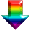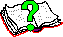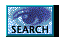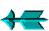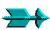\ -> PPWIZARD Extensions -> PPWSORT.H - Sorting

 PPWSORT.H - Sorting

PPWIZARD has an internal sort routine called ArraySort(), where possible it is recommended that it be used however the header file has the following advantages:

1. You can generate sort code inline when generating Rexx code (PPWIZARD's internal code will not be available when the generated code executes!).

2. You can sort more than one array at a time, this allows you to keep associated information together.

3. The compare operation can be as complex as you wish.

4. If you wish to sort an array with a known name (probably usual thing in PPWIZARD code) and there are many entries in the array then the sort can be much faster.

There are two generic macros you can choose from, "ArraySort" generates a PPWIZARD #evaluate command to perform the sort and "GenRexx2Sort" which generates the rexx code.

The first element in the array(s) is element 1 (not 0).

 The Generic Macro Parameters

1. COUNT
This is used to supply the number of elements that you are sorting.

2. PREFIX
By default the all variables used in the generated sort code are prefixed by "Srt" this parameter gives you the chance to remove any conflicts you may have (you may already have your own variables that begin with "Srt").

3. GREATER
You need to supply the rexx code which sets the "SrtGreater" variable to "1" if the first element is greater than the second or '0' otherwise. If the name of the array can't be hard coded (its name is held in a variable), the rexx value() routine will need to be used for all variable accesses.

4. SWAP
You need to supply the rexx code which swaps elements.

The last two macro parameters will need to use the "SrtIndex1" and "SrtIndex2" variables (using default prefix!) which hold the indexes of the elements to be compared or swapped.

 Some Non-Generic macros

While the generic macros above are very powerful (you can do anything after all) most people will probably never use them and use the macros described below (some of the same generic macro parameters are supported).

There are 2 macros which you can use to sort either 1 or 2 arrays (whose names are known - fixed). The default is that the ".0" element holds the number of items in the array and the sort is ascending. The macros are "GenRexx2Sort1Array" and "GenRexx2Sort2Arrays". With the 2 array sort macro the first array is sorted and the 2nd array is assumed to hold associated data.

There is one macros which you can use to sort a single array whose name is only known at runtime (not fixed), its name is "GenRexx2Sort1ArrayIndirect".

 EXAMPLES

This example shows the named array "Fred" being sorted:

```   <\$GenRexx2Sort1Array ARRAY="Fred">;
```

This time we want the first 4 items sorted in decending order:

```   <\$GenRexx2Sort1Array ARRAY="Fred" Compare="<" Count="4">;
```

We want a "ArraySort" routine in our generated rexx code:

```   ...
call ArraySort "fred";
return;
...
...
/*==========*/
ArraySort:
/*==========*/
ArrayNamedIn = translate(arg(1));  ;;Holds name of array

<\$GenRexx2Sort1ArrayIndirect NamedIn="ArrayNamedIn">;
return;
```

 PPWSORT.H

```;----------------------------------------------------------------------------
;     MODULE NAME:   PPWSORT.H
;
;         \$Author:   USER "Dennis"  \$
;       \$Revision:   1.0  \$
;           \$Date:   30 Mar 2001 18:05:30  \$
;        \$Logfile:   C:/DBAREIS/Projects.PVCS/MultiOs/PPWIZARD/ppwsort.h.pvcs  \$
;
;     DESCRIPTION:   PPWIZARD has a "ArraySort()" routine which can be used
;                    to sort a single array.  It does have some flexability
;                    in how it compares entries.
;
;                    This header file would be used to handle sort
;                    requirements that are not possible using "ArraySort()".
;
;                    This header allows you to:
;
;                         * Imbed the sort routine between PPWIZARD commands.
;
;                         * Imbed the sort routine between rexx     commands.
;
;                         * Sort more than one array at a time, for example
;                           you may have multiple arrays storing associated
;                           information that should all be sorted together.
;
;                         * Do any sort of compare, no matter how complex.
;
;
;                     EXAMPLE1 - Inline Rexx code (use "ArraySort" for inline
;                                PPWIZARD code). Array 'A' is being sorted.
;                     ~~~~~~~~~~~~~~~~~~~~~~~~~~~~~~~~~~~~~~~~~~~~~~~~~~~~~
;                     ArrayName = arg(1);      ;;Caller passed "A"
;                     <\$GenRexx2Sort                                                                                      \
;                          COUNT=^_valueG(ArrayName || '.0')^                                                             \
;                        GREATER=^SrtGreater = _valueG(ArrayName || '.SrtIndex1') >> _valueG(ArrayName || '.SrtIndex2')^  \
;                           SWAP=^SrtTemp = _valueG(ArrayName || '.SrtIndex1');                                          -\
;                                 call _valueS ArrayName || '.SrtIndex1', _valueG(ArrayName || '.SrtIndex2');            -\
;                                 call _valueS ArrayName || '.SrtIndex2', SrtTemp                                        -\
;                                ^                                                                                       -\
;                     >
;
;                     Note ">>" being used in the compare, if the data being
;                     sorted is numeric use '>' instead (or else)!!!!!!
;
;                     EXAMPLE2 - Exactly same as Example 1
;                                hardcoded - can't handle other arrays).
;                     ~~~~~~~~~~~~~~~~~~~~~~~~~~~~~~~~~~~~~~~~~~~~~~~~~~~~~
;                     ArrayName = translate(arg(1));      ;;Caller passed "A" (handle possible value() bug!)
;                     <\$GenRexx2Sort1ArrayIndirect NamedIn="ArrayName">
;
;                     EXAMPLE3 - In this case the name of the array ("A" is
;                                hardcoded - can't handle other arrays).
;                     ~~~~~~~~~~~~~~~~~~~~~~~~~~~~~~~~~~~~~~~~~~~~~~~~~~~~~
;                     <\$GenRexx2Sort1Array ARRAY="A">
;
;----------------------------------------------------------------------------

;--- Only include once ------------------------------------------------------
#ifndef VERSION_PPWSORT_H

;--- Define the version number of this header file --------------------------
#define   VERSION_PPWSORT_H    99.269
#require  99.269

;--- For use within PPWIZARD for immediate sorting of array (PPWIZARD Command!) ---
#define ArraySort    \
#evaluate '' ^<\$GenRexx2Sort COUNT={\$COUNT} GREATER={\$GREATER} SWAP={\$SWAP}>^

;--- Generates the rexx code to perform sorting (sort one "NAMED" array) ----
#define GenRexx2Sort1Array                                        \
<\$GenRexx2Sort                                            \
COUNT=^{\$Count="{\$Array}.0"}^                        \
PREFIX=^{\$Prefix="Srt"}^                              \
GREATER=^SrtGreater = {\$Array}.SrtIndex1 {\$Compare=">"} {\$Array}.SrtIndex2^  \
SWAP=^SrtTemp = {\$Array}.SrtIndex1;                -\
{\$Array}.SrtIndex1 = {\$Array}.SrtIndex2;     -\
{\$Array}.SrtIndex2 = SrtTemp                 -\
^                                             -\
>

;--- Generates the rexx code to perform sorting (sort 2 "NAMED" arrays) -----
#define GenRexx2Sort2Arrays                                                    \
<\$GenRexx2Sort                                                         \
COUNT=^{\$Count="{\$Array1}.0"}^                                    \
PREFIX=^{\$Prefix="Srt"}^                                           \
GREATER=^{\$Prefix}Greater = {\$Array1}.{\$Prefix}Index1 {\$Compare=">"} {\$Array1}.{\$Prefix}Index2^ \
SWAP=^{\$Prefix}Temp = {\$Array1}.{\$Prefix}Index1;                -\
{\$Array1}.{\$Prefix}Index1 = {\$Array1}.{\$Prefix}Index2;    -\
{\$Array1}.{\$Prefix}Index2 = {\$Prefix}Temp;                -\
{\$Prefix}Temp = {\$Array2}.{\$Prefix}Index1;                -\
{\$Array2}.{\$Prefix}Index1 = {\$Array2}.{\$Prefix}Index2;    -\
{\$Array2}.{\$Prefix}Index2 = {\$Prefix}Temp                 -\
^                                                          -\
>

;--- Generates the rexx code to perform sorting (array name not hard coded) -
#define GenRexx2Sort1ArrayIndirect                                                                             \
<\$GenRexx2Sort                                                                                         \
COUNT=^{\$Count="_valueG({\$NamedIn} || '.0')"}^                                                    \
PREFIX=^{\$Prefix="SRT"}^                                                                           \
GREATER=^{\$Prefix}Greater = _valueG({\$NamedIn} || '.{\$Prefix}INDEX1') {\$Compare=">"} _valueG({\$NamedIn} || '.{\$Prefix}INDEX2')^  \
SWAP=^{\$Prefix}Temp = _valueG({\$NamedIn} || '.{\$Prefix}INDEX1');                                -\
call _valueS {\$NamedIn} || '.{\$Prefix}INDEX1', _valueG({\$NamedIn} || '.{\$Prefix}INDEX2'); -\
call _valueS {\$NamedIn} || '.{\$Prefix}INDEX2', {\$Prefix}Temp                              -\
^                                                                                          -\
>

;--- Generates the rexx code to perform sorting (GENERIC) -------------------
#DefineRexx GenRexx2Sort
{\$Prefix="SRT"}M = 1
{\$Prefix}Count   = {\$COUNT};
do  while (9 * {\$Prefix}M + 4) < {\$Prefix}Count
{\$Prefix}M = {\$Prefix}M * 3 + 1
end /* do while */
do  while {\$Prefix}M > 0
{\$Prefix}K = {\$Prefix}Count - {\$Prefix}M
do  {\$Prefix}J = 1 to {\$Prefix}K
{\$Prefix}Index1 = {\$Prefix}J
do while {\$Prefix}Index1 > 0
;--- Work out what to compare it with ----------------
{\$Prefix}Index2 = {\$Prefix}Index1 + {\$Prefix}M;

;--- User user supplied logic to check for greater ---
{\$Greater};

;--- Swap if required --------------------------------
if {\$Prefix}Greater then
do
{\$Swap};
end;
else
leave;

;--- Prepare for the next loop -----------------------
{\$Prefix}Index1 = {\$Prefix}Index1 - {\$Prefix}M

end /* do while {\$Prefix}Index1 > 0 */
end     /* do {\$Prefix}J = 1 to {\$Prefix}K */
{\$Prefix}M = {\$Prefix}M % 3
end         /* while {\$Prefix}M > 0 */
#DefineRexx

#ifdef   CommentBlock  /* (Saturday 07/08/1999, 20:32:20, by Dennis_Bareis) */
**+--------------------------------------------------------------------------
**|;--- Generates the rexx code to perform sorting (GENERIC) -------------------
**|#DefineRexx GenRexx2Sort
**|        {\$Prefix="Srt"}Count    = {\$COUNT};
**|        {\$Prefix}Distance = {\$Prefix}Count % 2;
**|        do  while {\$Prefix}Distance > 0
**|            do  until {\$Prefix}Finished;      /* start of mini-bubblesort loop */
**|                {\$Prefix}Finished = 1;
**|                do  {\$Prefix}Index1 = 1 to {\$Prefix}Count - {\$Prefix}Distance
**|                    ;--- We now compare and swap items {\$Prefix}Index1 and {\$Prefix}Index1+{\$Prefix}Distance ---
**|                    {\$Prefix}Index2 = {\$Prefix}Index1 + {\$Prefix}Distance;
**|
**|                    ;--- User user supplied logic to check for greater ---
**|                    {\$GREATER};
**|
**|                    ;--- Swap if required --------------------------------
**|                    if {\$Prefix}Greater then
**|                    do
**|                        ;--- User user supplied logic to swap items ------
**|                        {\$Swap};
**|                        {\$Prefix}Finished = 0;
**|                    end;
**|                end;
**|            end;                       /* end of mini-bubblesort loop */
**|            {\$Prefix}Distance = {\$Prefix}Distance % 2;
**|        end;
**|#DefineRexx
**+--------------------------------------------------------------------------
#endif                 /* (Saturday 07/08/1999, 20:32:20, by Dennis_Bareis) */

#endif

```

Saturday May 28 2022 at 2:55pm